## RS Aggarwal Solutions Class 10 Chapter 18 Areas of Circle, Sector and Segment Ex 18b

These Solutions are part of RS Aggarwal Solutions Class 10. Here we have given RS Aggarwal Solutions Class 10 Chapter 18 Areas of Circle, Sector and Segment Ex 18b.

Other Exercises

Question 1.
Solution:
Circumference of circle = 2πr = 39.6 cm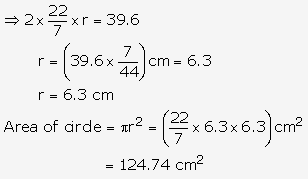Question 2.
Solution:Question 3.
Solution: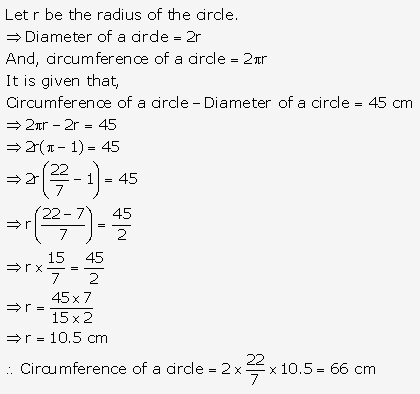Question 4.
Solution: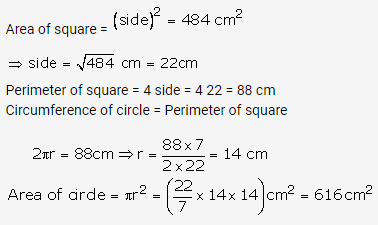Question 5.
Solution: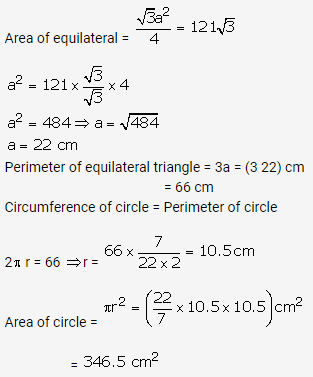Question 6.
Solution: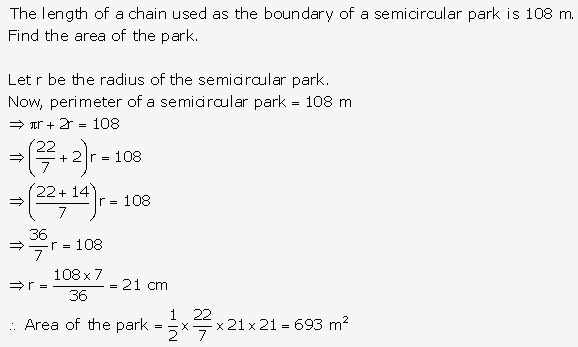Question 7.
Solution:
Let the radii of circles be x cm and (7 – x) cm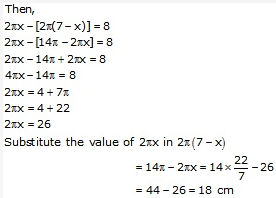Circumference of the circles are 26 cm and 18 cm.

Question 8.
Solution: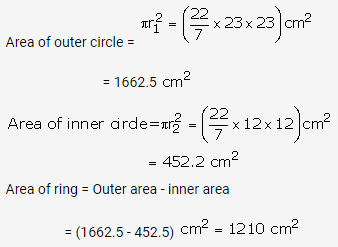Question 9.
Solution:
Inner radius of the circular park = 17 m
Width of the path = 8 m
Outer radius of the circular park = (17 + 8)m = 25 m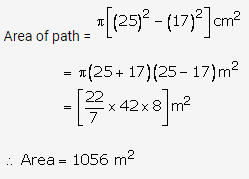Question 10.
Solution:
Let r m and R m be the radii of inner circle and outer boundaries respectively.
Then, 2πr = 352 and 2πR = 396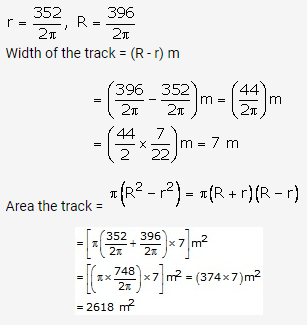Question 11.
Solution: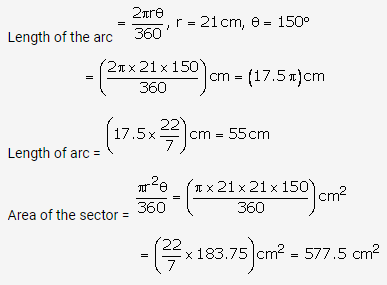Question 12.
Solution: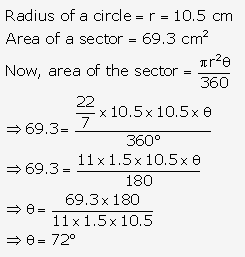Question 13.
Solution:Question 14.
Solution: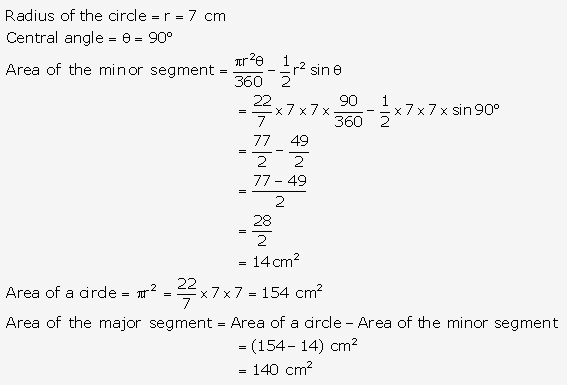Question 15.
Solution:
∆OAB is equilateral.
So, ∠AOB = 60Question 16.
Solution:Area of minor segment = (area of sector OACBO) – (area of ∆OAB)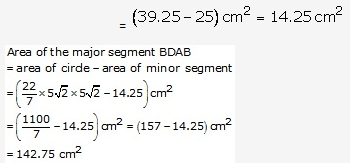Question 17.
Solution:
Area of sector OACBO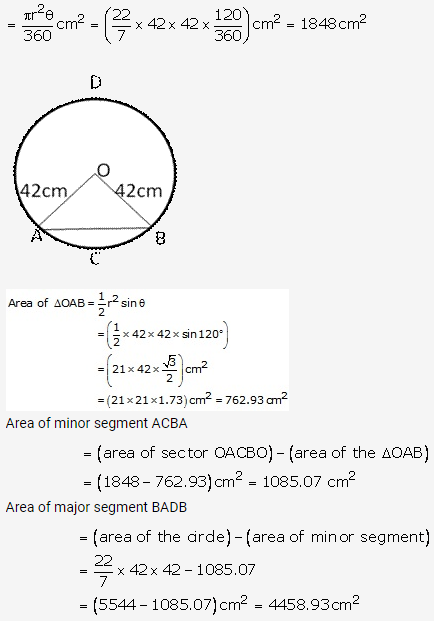Question 18.
Solution:
Let AB be the chord of circle of centre O and radius = 30 cm such that ∠AOB = 600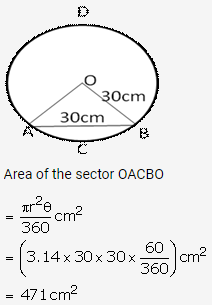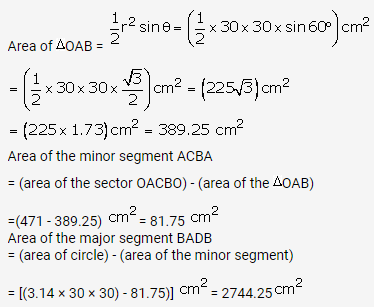Question 19.
Solution: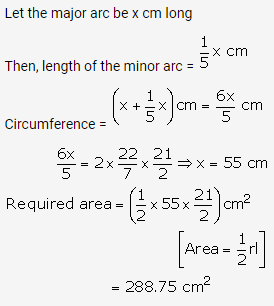Question 20.
Solution:
In 2 days, the short hand will complete 4 rounds
Distance travelled by its tip in 2 days
=4(circumference of the circle with r = 4 cm)
= (4 × 2π × 4) cm = 32π cm
In 2 days, the long hand will complete 48 rounds length moved by its tip
= 48(circumference of the circle with r = 6cm)
= (48 × 2π × 6) cm = 576π cm
Sum of the lengths moved
= (32π + 576π) = 608π cm
= (608 × 3.14) cm = 1909.12 cm

Question 21.
Solution: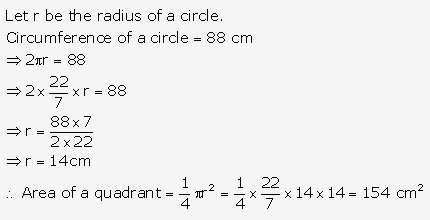Question 22.
Solution: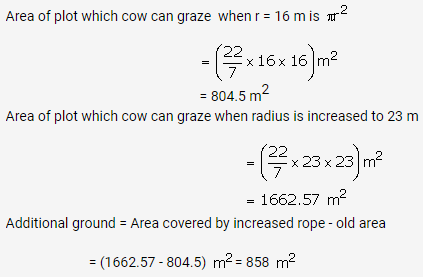Question 23.
Solution:Question 24.
Solution:
Each angle of equilateral triangle is 600Area that the horse cannot graze is 36.68 m2

Question 25.
Solution: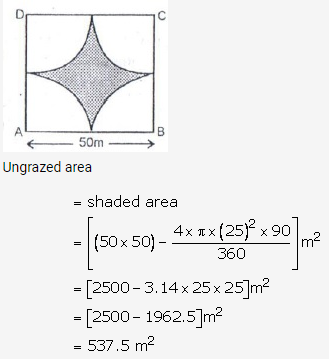Question 26.
Solution:OP = OR = OQ = r
Let OQ and PR intersect at S
We know the diagonals of a rhombus bisect each other at right angle.
Therefore we have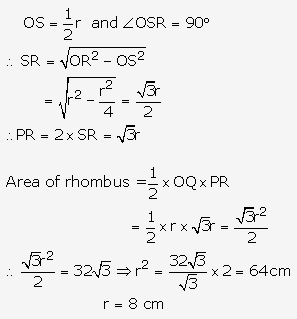Question 27.
Solution:
Diameter of the inscribed circle = Side of the square = 10 cm
Radius of the inscribed circle = 5 cm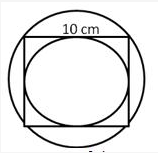Diameter of the circumscribed circle
= Diagonal of the square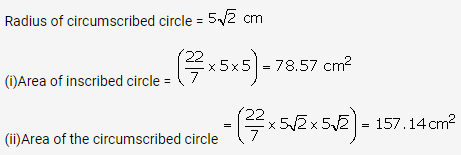Question 28.
Solution:
Let the radius of circle be r cm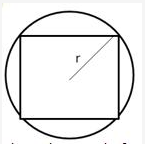Then diagonal of square = diameter of circle = 2r cm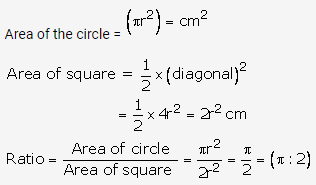Question 29.
Solution:
Let the radius of circle be r cmQuestion 30.
Solution:Question 31.
Solution:Question 32.
Solution: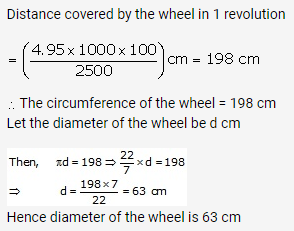Question 33.
Solution: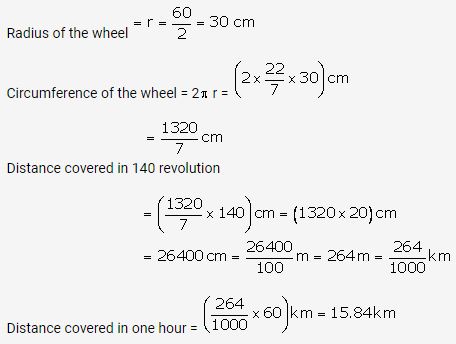Question 34.
Solution:
Distance covered by a wheel in 1minute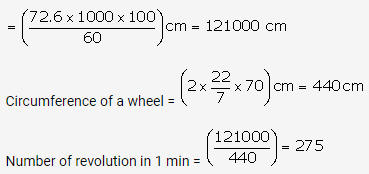Question 35.
Solution: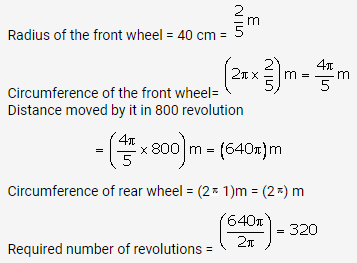Question 36.
Solution:
Each side of the square is 14 cm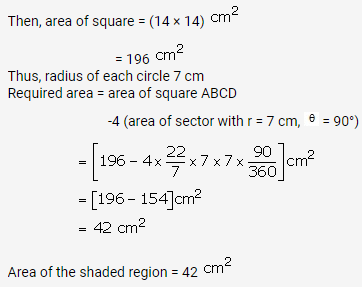Question 37.
Solution: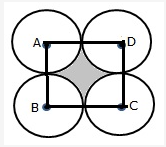Let A, B, C, D be the centres of these circles
Join AB, BC, CD and DA
Side of square = 10 cm
Area of square ABCD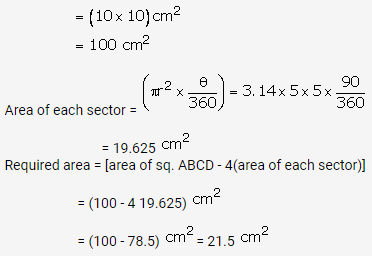Question 38.
Solution:Required area = [area of square – areas of quadrants of circles]
Let the side = 2a unit and radius = a units
Area of square = (side × side) = (2a × 2a) sq. units
= 4a2sq. units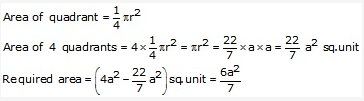Question 39.
Solution: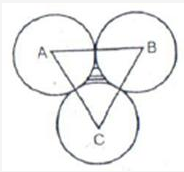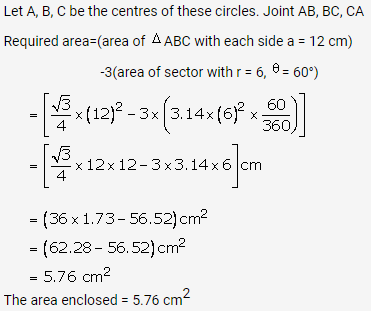Question 40.
Solution: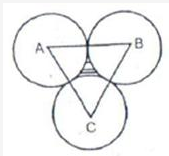Let A, B, C be the centers of these circles. Join AB, BC, CA
Required area= (area of ∆ABC with each side 2) -3[area of sector with r = a cm, θ= 600]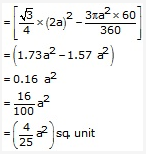Question 41.
Solution: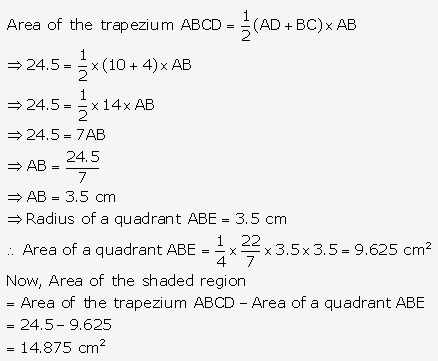Question 42.
Solution: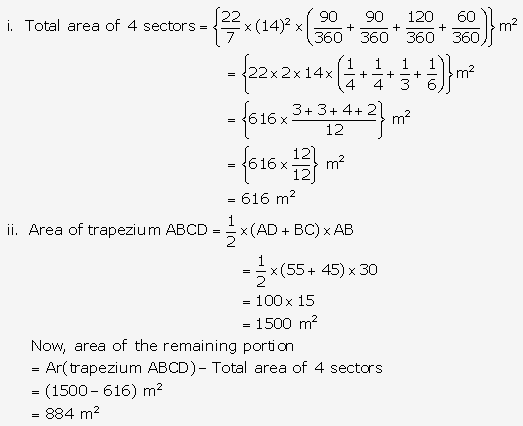Question 43.
Solution: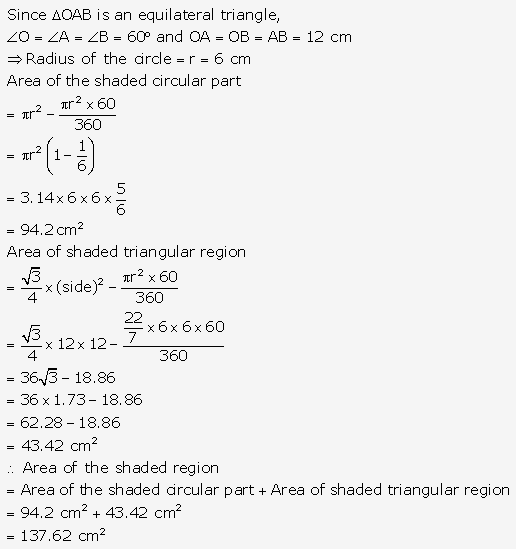Question 44.
Solution:Question 45.
Solution: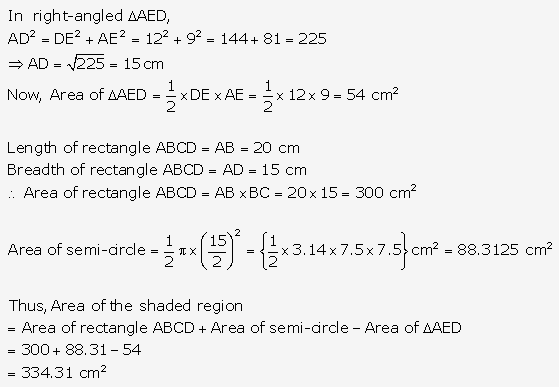Question 46.
Solution: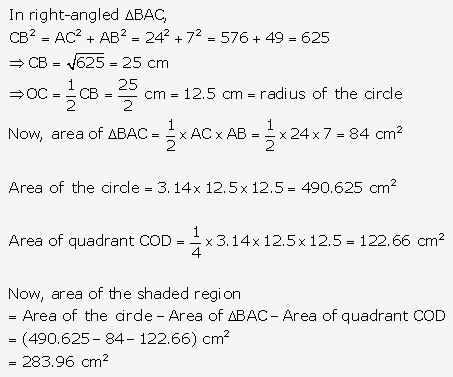Question 47.
Solution: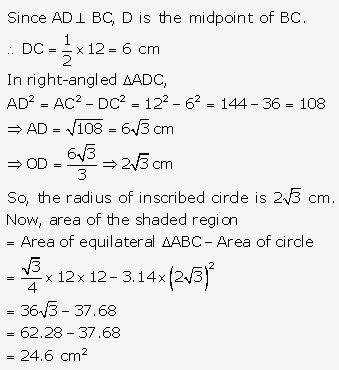Question 48.
Solution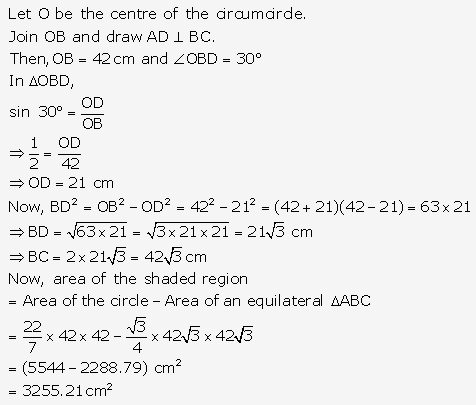Question 49.
Solution: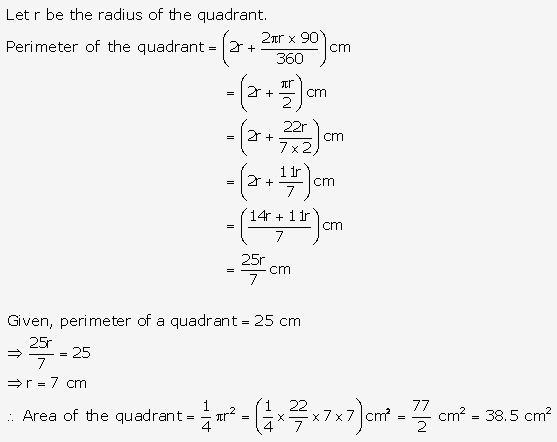Question 50.
Solution:Question 51.
Solution: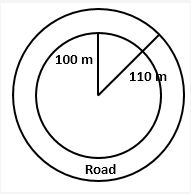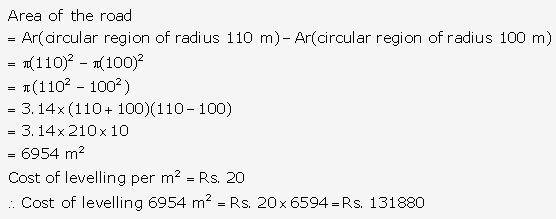Question 52.
Solution: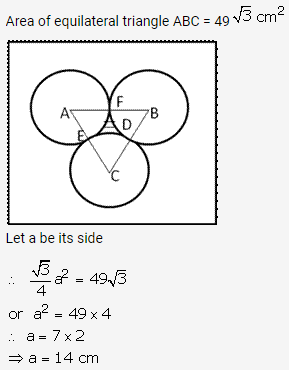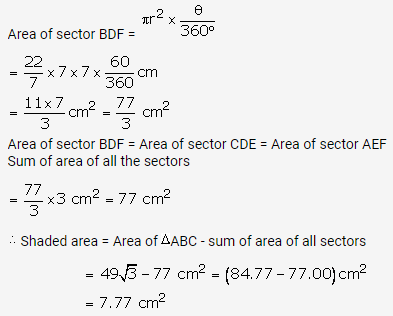Question 53.
Solution:Question 54.
Solution: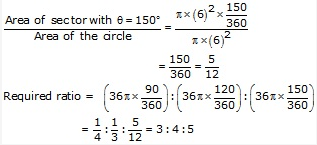Question 55.
Solution: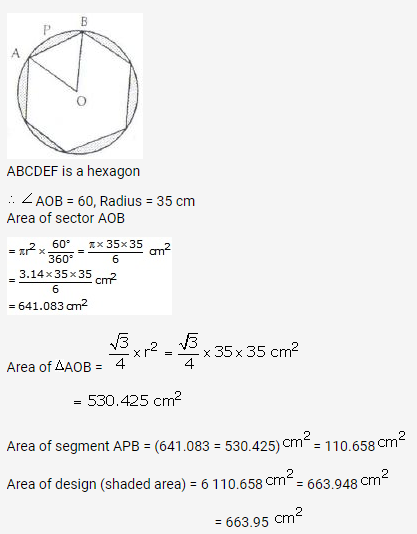Question 56.
Solution: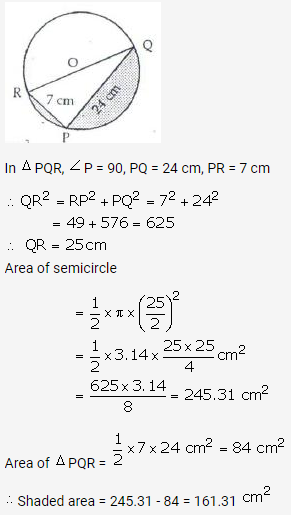Question 57.
Solution: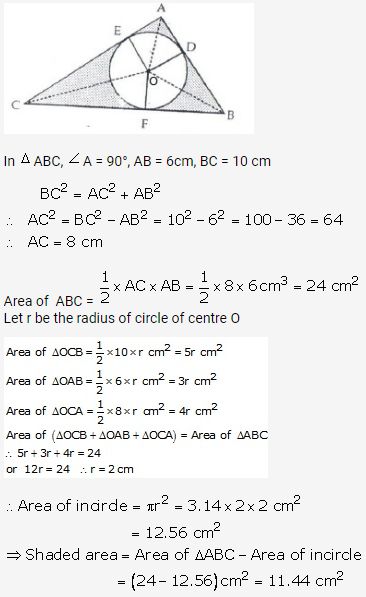Question 58.
Solution: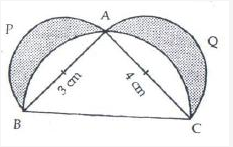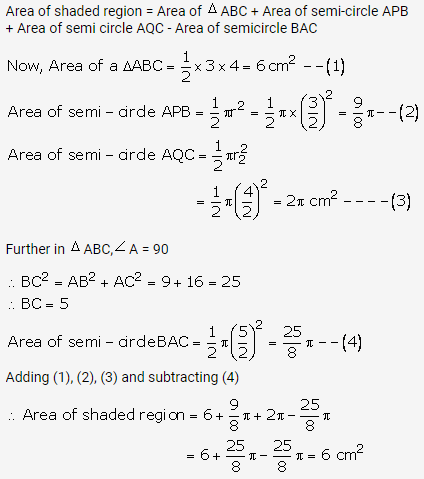Question 59.
Solution:
PS = 12 cm
PQ = QR = RS = 4 cm, QS = 8 cm
Perimeter = arc PTS + arc PBQ + arc QESQuestion 60.
Solution:Hope given RS Aggarwal Solutions Class 10 Chapter 18 Areas of Circle, Sector and Segment Ex 18b are helpful to complete your math homework.

If you have any doubts, please comment below. Learn Insta try to provide online math tutoring for you.